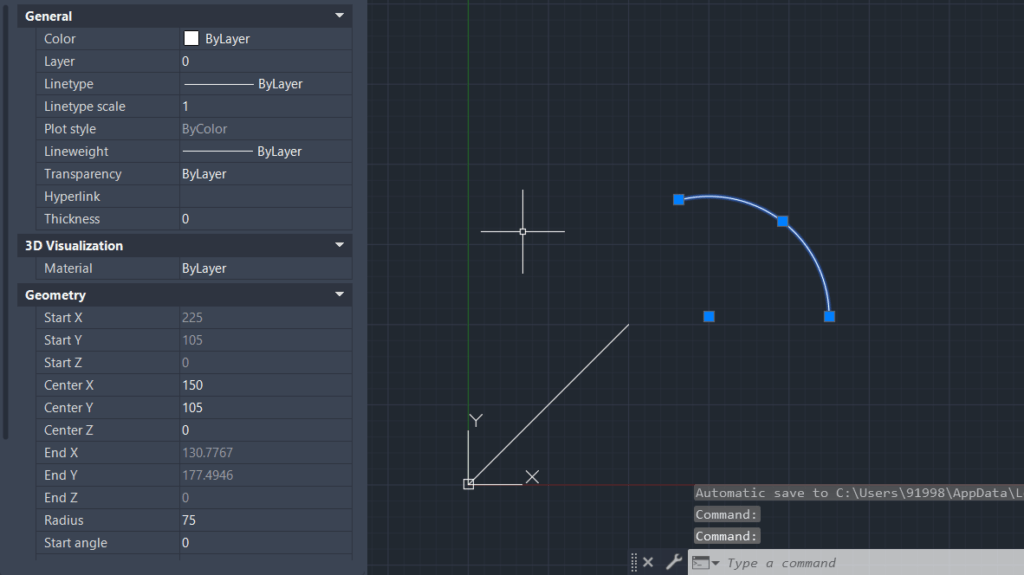In this blog post I will be discussing the IntersectWith method for dealing with intersecting objects in AutoCAD. The method can be used for identifying intersecting AutoCAD objects. It can also be used in combination with object extensions, i.e. when extending some AutoCAD object until it intersects with another AutoCAD object.

The IntersectMethod will prove to be one of the most widely used and most important pyautocad methods. Now, let me document this method for you in greater detail. This post will introduce the method and its parameters.

The “IntersectWith” method specifically helps us find intersecting objects based on a specified selection of criteria. Here is the syntax of the IntersectWith method:

``object.IntersectWith(IntersectObject, ExtendOption)``

From above syntax it is clear that the IntersectWith method is called for an AutoCAD object, and that the other object of the intersection is passed on as an input parameter. In addition, the ExtendOption parameter is meant for specifying specific criteria.

Here are the possible values which the ExtendOption parameter can take:

acExtendNone: Does not extend either object. This can fetch a 3D array of coordinate points if both the passed objects are already intersecting each other at a particular point. To use this parameter we have to pass 0 as parameter input value.

acExtendThisEntity: Extends the base object. If extending the object against which we are using the IntersectWith method is able to intersect the object we are passing as a parameter then this parameter gives us the point at which both the entities intersect after extending the first object. To use this parameter we need to pass 1 as parameter input value.

acExtendOtherEntity: Extends the object passed as an argument. Pass 2 as parameter input value if we want to fetch the point at which the two objects intersect on extending the object passed as a parameter.

acExtendBoth: Extends both objects. We need to pass 3 as parameter input value if we want to get the intersection point of two objects after extending both of them.

## IntersectWith method using acExtendNone parameter as ExtendOption

We will go through examples based on every criterion that we discussed earlier.

First, we will create 2 circles intersecting each other at two different points. Then we will check if the “IntersectWith” method passes us those two points.

``````c1 = acad.model.AddCircle(APoint(100, 100, 0), 75)

Let us apply the IntersectWith method against circle c1, and pass c2 as IntersectObject parameter. We can use it either way. Also, pass 0 for “acExtendNone” to check the intersection points.

``print(c1.IntersectWith(c2, 0))``

## IntersectWith method using acExtendThisEntity and acExtendOtherEntity parameters with pyautocad

Now I will move forward to the next criteria. This criteria is “acExtendThisEntity”. I try to extend an arc and a line and I then want to see if extending the objects in this way will result in an intersection or not.Figure 2.1: Drew arc and line to check if they intersect each other on extending either of them or not
``````a1 = acad.model.AddArc(APoint(150, 105, 0), 75, 0, round(105*pi/180,2))

print(l1.IntersectWith(a1, 1))``````

I can now simply pass the numeric value 2 as the ExtendOption parameter input value to check the intersection point when extending the arc further to meet the line from Figure 2.1.

I can verify whether the given output is correct from the property table in Figure 2.4

## IntersectWith method using acExtendBoth parameter with pyautocad

By now I can take a closer look at the final criteria, i.e. acExtendBoth.

I need to pass the numeric value 3 as a parameter input value for the ExtendOption. For instance, I now want to draw two lines and I will check at which point those lines meet if I was to extend both lines.

``````l1 = acad.model.AddLine(APoint(0, 0, 0), APoint(100, 100, 0))

print(l1.IntersectWith(l2, 3))``````

As the Python output suggests the two lines would cross, i.e. intersect, at x = 150, y = 150, z = 0.

## Empty tuple output

While working with the 4 parameters as discussed above, we might end up getting unexpected outputs.

For instance, we know that lines from Figure 3.1 can intersect each other only if we extend both of them.

Considering the same, we passed 3 as ExtendOption representing acExtendBoth. But if we would have passed any of the parameters from 0-2 it would result in an empty tuple.

Since we know that, extending only one of those two lines or neither of them won’t result in their intersection.

Let’s check the same, passing any of the parameters from 0-2.

``print(l1.IntersectWith(l2, 1))``

If you are interested in AutoCAD scriptin and automatization with Python, namely using pyautocad and pywin32, I advice you to check out the AutoCAD section of this blog. Subject that I have covered also comprise e.g.:

• Creating and modifying 3D mesh objects with pyautocad
• Integrating Python with AutoCAD using pywin32
• Working with pollar arrays with pyautocad

1.Nuwan says:
1.Tanmay Sawant says: